• 写文章不易，如果您觉得此文对您有所帮助，请帮忙点赞、评论、收藏，感谢您！ 一. 仿射变换介绍：  请参考：图解图像仿射变换... python实现仿射变换——图像平移 import cv2 import numpy as np # 图像仿射变换->图
• 主要介绍了Python 在OpenCV里实现仿射变换—坐标变换效果，本文通过一个例子给大家介绍的非常详细，具有一定的参考借鉴价值,需要的朋友可以参考下
• 一般对图像的变化操作有放大、缩小、旋转等，统称为几何变换，对一个图像的图像变换主要有两大步骤，一是实现空间坐标...仿射变换##二维空间坐标的仿射变换公式：$\left(\begin{matrix}\overline{x} \\\overline{y... 一般对图像的变化操作有放大、缩小、旋转等，统称为几何变换，对一个图像的图像变换主要有两大步骤，一是实现空间坐标的转换，就是使图像从初始位置到终止位置的移动。二是使用一个插值的算法完成输出图像的每个像素的灰度值。其中主要的图像变换有：仿射变换、投影变换、极坐标变换。 仿射变换## 二维空间坐标的仿射变换公式： \[\left( \begin{matrix} \overline{x} \\ \overline{y} \end{matrix} \right) =\left( \begin{matrix} a_{11} &&a_{12} \\ a_{21}&&a_{22} \end{matrix} \right) \left( \begin{matrix} x \\ y \end{matrix} \right) + \left( \begin{matrix} a_{13} \\ a_{23} \end{matrix} \right)$

在以下矩阵中：

$\left( \begin{matrix} \overline{x} \\ \overline{y} \\ 1 \end{matrix} \right) =A \left( \begin{matrix} x \\ y \\ 1 \end{matrix} \right)$

矩阵A就是仿射矩阵，因为它最后一行为(0，0，1)

$A= \left( \begin{matrix} a_{11}&a_{12}&a_{13} \\ a_{21}&a_{22}&a_{23} \\ 0&0&1 1 \end{matrix} \right)$

平移

平移是最简单的仿射变换如将空间坐标(x,y)沿着x轴移动100，沿着y轴移动200。平移后的坐标为(x+100,y+200)。将这个过程一般化后，假设任意的空间坐标(x,y)先沿着x轴平移Px再沿着y轴平移Py。得到的坐标为(x+Px,y+Py)。用矩阵表示这个平移过程为：

$\left( \begin{matrix} \overline{x} \\ \overline{y} \\ 1 \end{matrix} \right) =\left( \begin{matrix} 1 &0&Px \\ 0&1&Py \\ 0&0&1 \end{matrix} \right) \left( \begin{matrix} x \\ y \\ 1 \end{matrix} \right)$

对于Px和Py若大于0则表示沿着轴正向移动，若小于0则表示沿着轴负向移动。

放大缩小

在坐标轴中以原点为中心的放大与缩小S倍是指对其x轴方向的横坐标放缩成原坐标的横坐标距离中心点(0，0)的距离的S倍并对其y轴方向的横坐标放缩成原坐标的纵坐标距离原点的距离的S倍。其中若S大于1则表示增大，若小于1则表示缩小。放缩在矩阵中的表示为：

$\left( \begin{matrix} \overline{x} \\ \overline{y} \\ 1 \end{matrix} \right) =\left( \begin{matrix} s_x&0&0 \\ 0&s_y&0 \\ 0&0&1 \end{matrix} \right) \left( \begin{matrix} x \\ y \\ 1 \end{matrix} \right)$

坐标(x,y)在坐标轴中以任意一点的坐标(x0,y0)为中心在水平和垂直方向上放缩S倍，放缩后的坐标为$$( (x_0+S_x(x-x_0),y_0+S_y(y-y_0) )$$用矩阵可以表示为：

$\left( \begin{matrix} \overline{x} \\ \overline{y} \\ 1 \end{matrix} \right) =\left( \begin{matrix} 1 &0&X_0 \\ 0&1&Y_0 \\ 0&0&1 \end{matrix} \right) \left( \begin{matrix} s_x&0&0 \\ 0&s_y&0 \\ 0&0&1 \end{matrix} \right) \left( \begin{matrix} 1 &0&-X_0 \\ 0&1&-Y_0 \\ 0&0&1 \end{matrix} \right) \left( \begin{matrix} x \\ y \\ 1 \end{matrix} \right)$

坐标(x,y)绕原点顺时针旋转α(α>0)，cosΘ=x/p sinΘ=y/p.其中p代表(x,y)到中心点(0,0)的距离。则

cos(Θ+α)=cosΘcosα-sinΘsinα=(x/p)cosα -(y/p)sinα=Ex/p

sin(Θ+α)=sinΘcosα+cosΘsinα=(y/p)cosα -(y/p)sinα=Ey/p

化解以上公式，使用矩阵表示为：

$\left( \begin{matrix} \overline{x} \\ \overline{y} \\ 1 \end{matrix} \right) =\left( \begin{matrix} cosα&-sinα&0 \\ sinα&cosα&0 \\ 0&0&1 \end{matrix} \right) \left( \begin{matrix} x \\ y \\ 1 \end{matrix} \right)$

放射矩阵的计算

如果已知坐标以及其放射变换后的矩阵，从而计算出变换后的坐标，就需要放射矩阵的计算，主要的实现方法有：方程法，矩阵法，插值算法。在OpenCV中有对应的实现函数，如使用方程法：cv2.getAffineTransform(src,dst) 该方法就是通过计算参数src到dst的对应仿射变换的矩阵，其中参数src和dst分别代表原坐标和变换后的坐标，并且均为3行2列的二维ndarray,数据必须为浮点型。实现代码：

import numpy as np

src=np.array([[0,0],[200,0],[0,200]],np.float32)

dst=np.array([[0,0],[100,0],[0,100]],np.float32)

A=cv2.getAffineTransform(src,dst)

print(A)

运行结果：在矩阵法中，需要预先知道具体的变化步骤，比如先放大再平移还是先移动再放大

$\left( \begin{matrix} \overline{x} \\ \overline{y} \\ 1 \end{matrix} \right) =\left( \begin{matrix} 1 &0&X_0 \\ 0&1&Y_0 \\ 0&0&1 \end{matrix} \right) \left( \begin{matrix} s_x&0&0 \\ 0&s_y&0 \\ 0&0&1 \end{matrix} \right) \left( \begin{matrix} x \\ y \\ 1 \end{matrix} \right)$

以上的矩阵变换就是平移仿射矩阵乘以缩放仿射矩阵得到的而不是缩放仿射矩阵乘以平移仿射矩阵得到的，由于等式是由右向左运行，所以必须要知道变化顺序。矩阵的乘法并不是矩阵的点乘，再Numpy中乘法是通过dot函数实现的，关于Numpy语法可以参考我之前写的博文。我们通过一个实例来了解矩阵的乘法计算。

假设先对一矩阵等比例放大二倍，然后水平与垂直方向上分别平移100，计算该矩阵的算法如下：

import numpy as np

#先对矩阵进行放大

s=np.array([[2,0,0],[0,2,0],[0,0,1]])

#再对矩阵进行平移

t=np.array([[1,0,100],[0,1,100],[0,0,1]])

#矩阵相乘

A=np.dot(t,s)

print(A)

运行结果：一定要注意传入dot参数的顺序。

下面介绍插值算法，我们可以将图像理解为一个二维的函数，行数为H，列数为W的图像矩阵I：Z=F(x,y), 0<=x

矩阵的列号对应x坐标，垂直方向为y轴，行号对应y坐标，称该函数为图像函数。

利用已知的整数坐标处的函数值估算非整数坐标处的函数值的方法主要有：最近邻插值(就是从四个相邻整数坐标中找到一个最近的)输出的图像经过放大后会有锯齿状的外观。双线性插值法有两个变量的插值函数的线性插值扩展，其核心是在两个方向分别进行一次线性插值。待插点像素值取原图像中与其相邻的４个点像素值的水平、垂直两个方向上的线性内插，即根据待采样点与周围４个邻点的距离确定相应的权重，从而计算出待采样点的像素值。有的时候需要更高阶的插值函数，如三次样条插值、Lengendre中心函数和sin(axs)函数，高阶插值常用二维离散卷积运算来实现。后续博客会对二维离散卷积运算做详细的描述。

对于双线性插值法在这表一次形象化的描述：如图：先估计f1在(x,[y])处的函数值，再估计f1在(x,[y]+1)处的函数值，最后估计f1在(x,y)处的函数值

对于空间坐标变换和插值方法在已知的仿射变换矩阵上OpenV提供了warpAffine(src,M,dsize[,flags[,borderMode[,borderValue ]]])函数

参数

释义

src

图像矩阵

M

2行3列的仿射变换矩阵

dsize

一个二元元组，输出图像的大小

flags

插值法：INTE_NEAREST、INTE_LINEAR(默认)等

borderMode

填充模式，如：BORDER_CONSTANT等

borderValue

当borderMode=BORDER_CONSTANT时的填充值

下面使用python实现图像的几何变换：

import numpy as np

import cv2

import sys

import math

cv2.imwrite('yun.jpg',img)

#原图的宽高

h,w=img.shape[:2]

#仿射变换矩阵 缩小2倍

A1=np.array([[0.5,0,0],[0,0.5,0]],np.float32)

A2=cv2.warpAffine(img,A1,(w,h),borderValue=126)

#缩小后平移

B1=np.array([[0.5,0,w/4],[0,0.5,h/4]],np.float32)

B2=cv2.warpAffine(img,B1,(w,h),borderValue=126)

#使图像旋转

C1=cv2.getRotationMatrix2D((w/2.0,h/2.0),30,1)

C2=cv2.warpAffine(img,C1,(w,h),borderValue=126)

cv2.imshow('img',img)

cv2.imshow('A2',A2)

cv2.imshow('B2',B2)

cv2.imshow('C2',C2)

cv2.waitKey(0)

cv2.destroyAllWindows()

运行效果参考文献：

ROBERT G.KEYS.Cubic Convolution Interpolation for Digital Image Processing.IEEE TRANSACTIONS ON ACOUSTICS.SPEECH,AND SIGNAL PROCESSING,1981

R. Hartley and A. Zisserman, “Multiple View Geometry in Computer Vision,” 2-nd edition, Cambridge University Press, 2004.

今天就先写到这吧！投影变换和极坐标变换后续再写

展开全文• 图像仿射变换python实现

千次阅读 2020-03-18 21:54:21
写文章不易，如果您觉得此... 仿射变换介绍： 请参考：图解图像仿射变换：https://www.cnblogs.com/wojianxin/p/12518393.html 图像仿射变换之图像平移：https://www.cnblogs.com/wojianxin/p/12519498.html 二...

写文章不易，如果您觉得此文对您有所帮助，请帮忙点赞、评论、收藏，感谢您！

一. 仿射变换介绍：

请参考：图解图像仿射变换：https://www.cnblogs.com/wojianxin/p/12518393.html

二. 仿射变换 公式：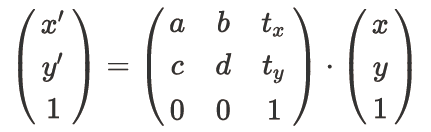仿射变换过程，(x,y)表示原图像中的坐标，(x',y')表示目标图像的坐标 ↑

三. 实验：利用我的上一篇文章（https://www.jianshu.com/p/1cfb3fac3798）的算法实现图像仿射变换——图像缩放

要实现其他功能的仿射变换，请读者照葫芦画瓢，自行举一反三：

实验目标，将输入图像在x方向上放大至原来的1.5倍，在y方向上缩小为原来的0.6倍。并沿x轴负向移动30像素，y轴正向移动100像素。

实验代码：

import cv2
import numpy as np

# Affine Transformation
def affine(img, a, b, c, d, tx, ty):
H, W, C = img.shape

# temporary image
tem = img.copy()
img = np.zeros((H+2, W+2, C), dtype=np.float32)
img[1:H+1, 1:W+1] = tem

# get new image shape
H_new = np.round(H * d).astype(np.int)
W_new = np.round(W * a).astype(np.int)
out = np.zeros((H_new+1, W_new+1, C), dtype=np.float32)

# get position of new image
x_new = np.tile(np.arange(W_new), (H_new, 1))
y_new = np.arange(H_new).repeat(W_new).reshape(H_new, -1)

# get position of original image by affine
adbc = a * d - b * c
x = np.round((d * x_new  - b * y_new) / adbc).astype(np.int) - tx + 1
y = np.round((-c * x_new + a * y_new) / adbc).astype(np.int) - ty + 1

x = np.minimum(np.maximum(x, 0), W+1).astype(np.int)
y = np.minimum(np.maximum(y, 0), H+1).astype(np.int)

# assgin pixcel to new image
out[y_new, x_new] = img[y, x]

out = out[:H_new, :W_new]
out = out.astype(np.uint8)

return out

# Affine
out = affine(image, a=1.5, b=0, c=0, d=0.6, tx=-30, ty=100)
# Save result
cv2.imshow("result", out)
cv2.imwrite("out.jpg", out)
cv2.waitKey(0)
cv2.destroyAllWindows()

四. 实验中的难点，晦涩难懂的代码讲解：

五. 实验结果：原图 ↑仿射变换结果(x*1.5-30,y*0.6+100) ↑

六. 参考文献：

七. 版权声明：

未经作者允许，请勿随意转载抄袭，抄袭情节严重者，作者将考虑追究其法律责任，创作不易，感谢您的理解和配合！

展开全文数字图像处理 仿射变换 python实现 图像缩放
• 仿射变换 公式：仿射变换过程，(x,y)表示原图像中的坐标，(x',y')表示目标图像的坐标 ↑要实现其他功能的仿射变换，请读者照葫芦画瓢，自行举一反三：实验目标，将输入图像在x方向上放大至原来的1.5倍，在y方向上...

写文章不易，如果您觉得此文对您有所帮助，请帮忙点赞、评论、收藏，感谢您！

一. 仿射变换介绍：

二. 仿射变换 公式：仿射变换过程，(x,y)表示原图像中的坐标，(x',y')表示目标图像的坐标 ↑

要实现其他功能的仿射变换，请读者照葫芦画瓢，自行举一反三：

实验目标，将输入图像在x方向上放大至原来的1.5倍，在y方向上缩小为原来的0.6倍。并沿x轴负向移动30像素，y轴正向移动100像素。

实验代码：

import cv2

import numpy as np

# Affine Transformation

def affine(img, a, b, c, d, tx, ty):

H, W, C = img.shape

# temporary image

tem = img.copy()

img = np.zeros((H+2, W+2, C), dtype=np.float32)

img[1:H+1, 1:W+1] = tem

# get new image shape

H_new = np.round(H * d).astype(np.int)

W_new = np.round(W * a).astype(np.int)

out = np.zeros((H_new+1, W_new+1, C), dtype=np.float32)

# get position of new image

x_new = np.tile(np.arange(W_new), (H_new, 1))

y_new = np.arange(H_new).repeat(W_new).reshape(H_new, -1)

# get position of original image by affine

adbc = a * d - b * c

x = np.round((d * x_new  - b * y_new) / adbc).astype(np.int) - tx + 1

y = np.round((-c * x_new + a * y_new) / adbc).astype(np.int) - ty + 1

x = np.minimum(np.maximum(x, 0), W+1).astype(np.int)

y = np.minimum(np.maximum(y, 0), H+1).astype(np.int)

# assgin pixcel to new image

out[y_new, x_new] = img[y, x]

out = out[:H_new, :W_new]

out = out.astype(np.uint8)

return out

# Affine Transformation

out = affine(image, a=1.5, b=0, c=0, d=0.6, tx=-30, ty=100)

# Save result

cv2.imshow("result", out)

cv2.imwrite("out.jpg", out)

cv2.waitKey(0)

cv2.destroyAllWindows()

四. 实验中的难点，晦涩难懂的代码讲解：

五. 实验结果：原图 ↑仿射变换结果(x*1.5-30,y*0.6+100) ↑

六. 参考文献：

七. 版权声明：

未经作者允许，请勿随意转载抄袭，抄袭情节严重者，作者将考虑追究其法律责任，创作不易，感谢您的理解和配合！

展开全文• 图像的仿射变换原理及python实现

万次阅读 多人点赞 2018-09-21 13:16:17
1. 原理 ...仿射变换保持了二维图形的“平直性”（直线经仿射变换后依然为直线）和“平行性”（直线之间的相对位置关系保持不变，平行线经仿射变换后依然为平行线，且直线上点的位置顺序不会发生变化）...

1. 原理

1.1 原理

仿射变换（Affine Transformation 或Affine Map）是一种二维坐标（x, y）到二维坐标（u, v）的线性变换，其数学表达式形式如下：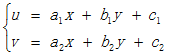对应的齐次坐标矩阵表示形式为：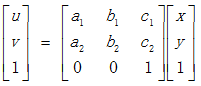仿射变换保持了二维图形的“平直性”（直线经仿射变换后依然为直线）和“平行性”（直线之间的相对位置关系保持不变，平行线经仿射变换后依然为平行线，且直线上点的位置顺序不会发生变化）。非共线的三对对应点确定一个唯一的仿射变换。

1.2 二维图像仿射变换

图像处理中，可应用仿射变换对二维图像进行平移、缩放、旋转等操作。实例如下：经仿射变换后，图像关键点依然构成三角形，但三角形形状已经发生变化。

1.3 原子变换

仿射变换通过一系列原子变换复合实现，具体包括：平移（Translation）、缩放（Scale）、旋转（Rotation）、翻转（Flip）和错切（Shear）。
a. 平移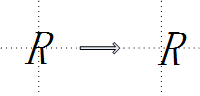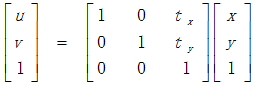b. 缩放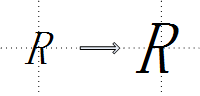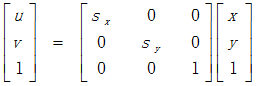c. 旋转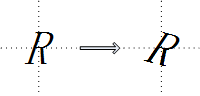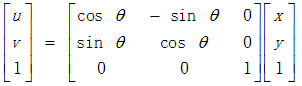d. 翻转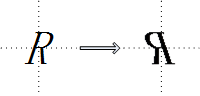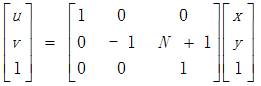e. 错切
错切亦称为剪切或错位变换，包含水平错切和垂直错切，常用于产生弹性物体的变形处理。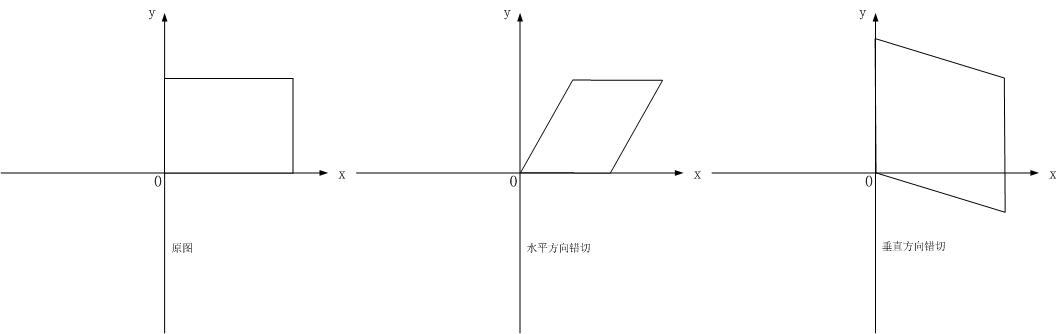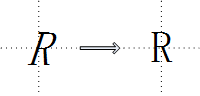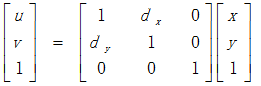1.4 程序

clc;
clear all;close all;
clc;

% u = 4 * x and v = 2 * y
T = [4 0 0; 0 2 0; 0 0 1];

% create spatial transformation structure.
transformation = maketform('affine', T);

% apply 2D spatial transformation to image.
transformationResult = imtransform(image, transformation);

结果示例：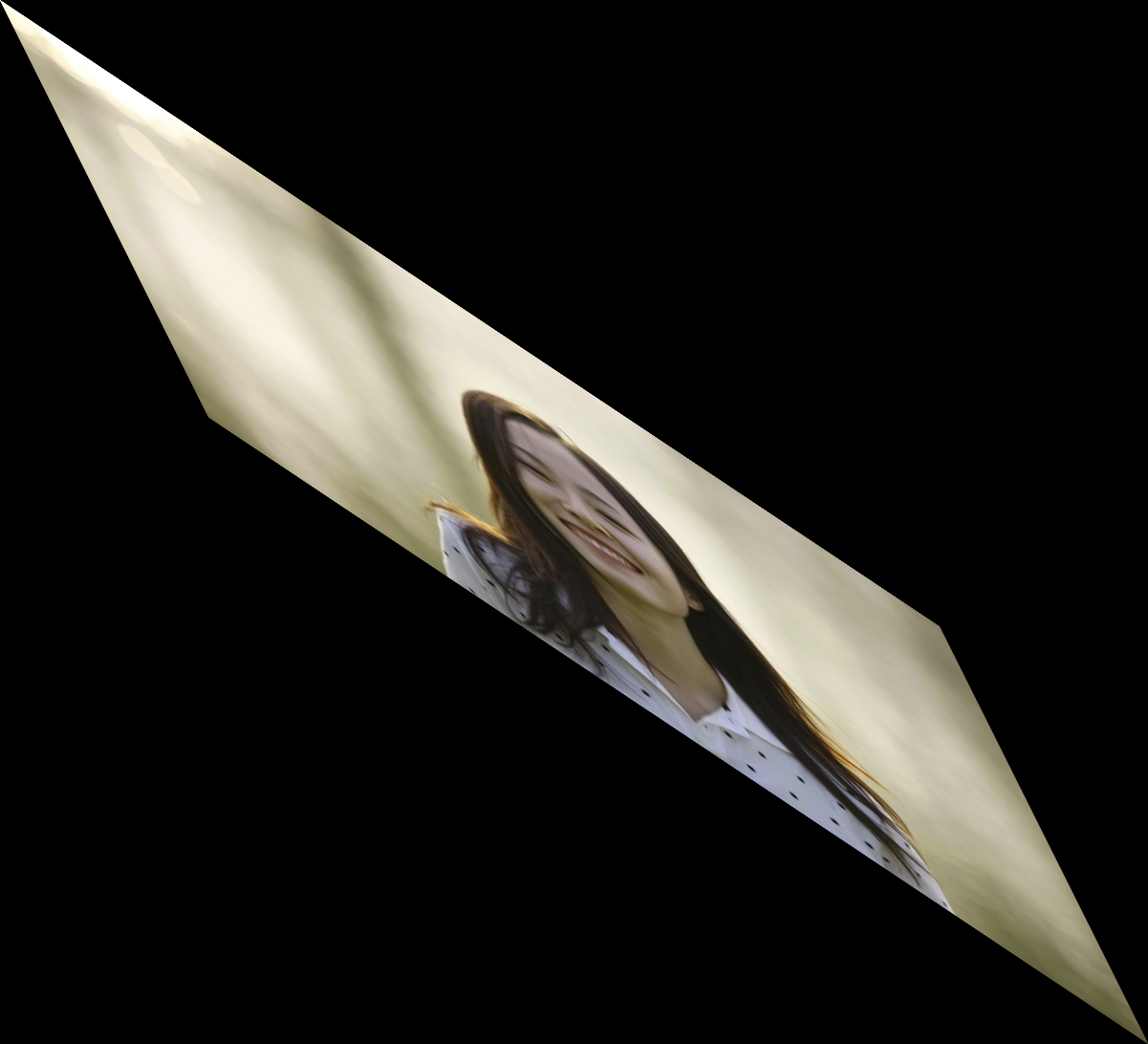2. Python-opencv实现图像的几何变换

2.1 写在前面

二维与三维图像的几何变换在计算机图形学上有重要的应用，包括现在的许多图像界面的切换、二维与三维游戏画面控制等等都涉及到图像几何变换，就比如说在三维游戏中，控制角色三维移动的时候，画面是要跟着移动的，那么怎么移动，怎么让上一时刻的画面移动到这一时刻，这都是根据了你的移动量，然后找到三维坐标之间的对应关系，用这一时刻的坐标替换到上一时刻的坐标像素值实现图像的切换。
图像的几何变换主要包括：平移、扩大与缩小、旋转、仿射、透视等等。图像变换是建立在矩阵运算基础上的，通过矩阵运算可以很快的找到对应关系。理解变换的原理需要理解变换的构造方法以及矩阵的运算方法，曾经写过matlab下的简单图像变换原理，里面有最基础的构造原理可以看看： matlab之原始处理图像几何变换

2.2 图像的平移

下面介绍的图像操作假设你已经知道了为什么需要用矩阵构造才能实现了（上面那个博客有介绍为什么）。那么关于偏移很简单，图像的平移，沿着x方向tx距离，y方向ty距离，那么需要构造移动矩阵：

通过numpy来产生这个矩阵，并将其赋值给仿射函数cv2.warpAffine().
仿射函数cv2.warpAffine()接受三个参数，需要变换的原始图像，移动矩阵M 以及变换的图像大小（这个大小如果不和原始图像大小相同，那么函数会自 动通过插值来调整像素间的关系）。
一个例子如下：

import cv2
import numpy as np
import matplotlib.pyplot as plt

H = np.float32([[1,0,100],[0,1,50]])
rows,cols = img.shape[:2]
res = cv2.warpAffine(img,H,(rows,cols)) #需要图像、变换矩阵、变换后的大小
plt.subplot(121)
plt.imshow(img)
plt.subplot(122)
plt.imshow(res)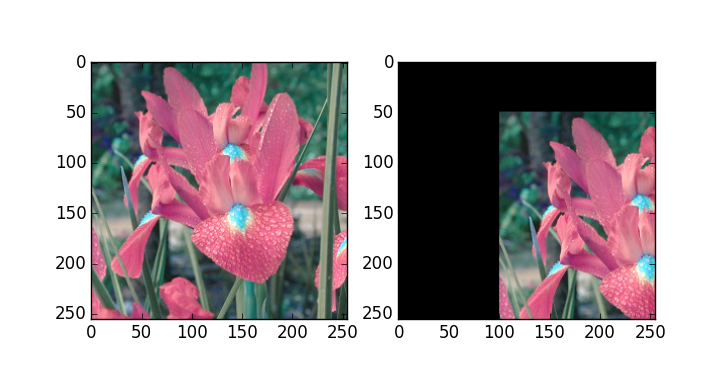2.3 图像的扩大与缩小

图像的扩大与缩小有专门的一个函数，cv2.resize()，那么关于伸缩需要确定的就是缩放比例，可以是x与y方向相同倍数，也可以单独设置x与y的缩放比例。另外一个就是在缩放以后图像必然就会变化，这就又涉及到一个插值问题。那么这个函数中，缩放有几种不同的插值（interpolation）方法，在缩小时推荐cv2.INTER_ARER,扩大是推荐cv2.INTER_CUBIC和cv2.INTER_LINEAR。默认都是cv2.INTER_LINEAR，比如：

import cv2
import matplotlib.pyplot as plt

# 插值：interpolation
# None本应该是放图像大小的位置的，后面设置了缩放比例，
#所有就不要了
res1 = cv2.resize(img,None,fx=2,fy=2,interpolation=cv2.INTER_CUBIC)
#直接规定缩放大小，这个时候就不需要缩放因子
height,width = img.shape[:2]
res2 = cv2.resize(img,(2*width,2*height),interpolation=cv2.INTER_CUBIC)
plt.subplot(131)
plt.imshow(img)
plt.subplot(132)
plt.imshow(res1)
plt.subplot(133)
plt.imshow(res2)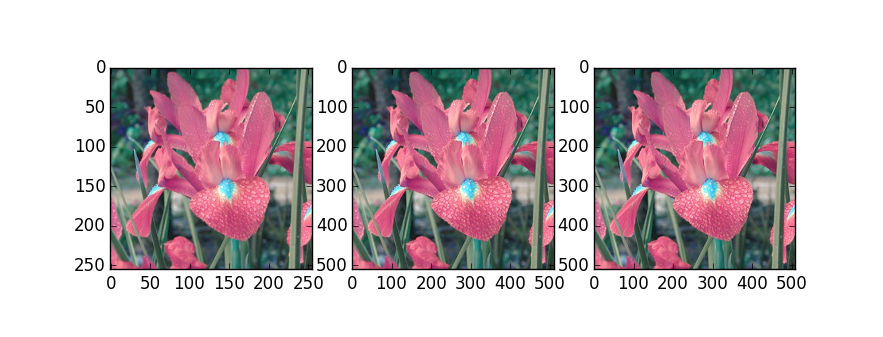通过坐标轴可以看到图像扩大了一倍，并且两种方法相同。

2.4 图像的旋转

图像的旋转矩阵一般为：但是单纯的这个矩阵是在原点处进行变换的，为了能够在任意位置进行旋转变换，opencv采用了另一种方式：

为了构造这个矩阵，opencv提供了一个函数：
cv2.getRotationMatrix2D()，这个函数需要三个参数，旋转中心，旋转角度，旋转后图像的缩放比例，比如下例：

import cv2
import matplotlib.pyplot as plt

rows,cols = img.shape[:2]
#第一个参数旋转中心，第二个参数旋转角度，第三个参数：缩放比例
M = cv2.getRotationMatrix2D((cols/2,rows/2),45,1)
#第三个参数：变换后的图像大小
res = cv2.warpAffine(img,M,(rows,cols))

plt.subplot(121)
plt.imshow(img)
plt.subplot(122)
plt.imshow(res)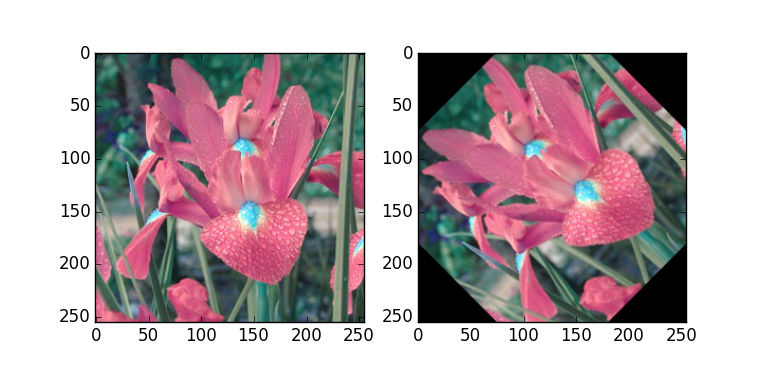2.5 图像的仿射

图像的旋转加上拉升就是图像仿射变换，仿射变化也是需要一个M矩阵就可以，但是由于仿射变换比较复杂，一般直接找很难找到这个矩阵，opencv提供了根据变换前后三个点的对应关系来自动求解M。这个函数是
M=cv2.getAffineTransform(pos1,pos2),其中两个位置就是变换前后的对应位置关系。输 出的就是仿射矩阵M。然后在使用函数cv2.warpAffine()。形象化的图如下（引用参考的）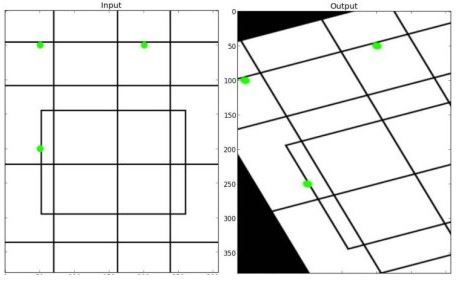一个例子比如：

import cv2
import numpy as np
import matplotlib.pyplot as plt

rows,cols = img.shape[:2]
pts1 = np.float32([[50,50],[200,50],[50,200]])
pts2 = np.float32([[10,100],[200,50],[100,250]])
M = cv2.getAffineTransform(pts1,pts2)
#第三个参数：变换后的图像大小
res = cv2.warpAffine(img,M,(rows,cols))
plt.subplot(121)
plt.imshow(img)
plt.subplot(122)
plt.imshow(res)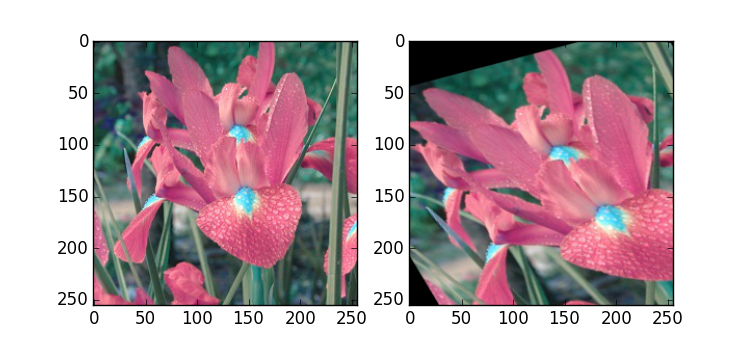2.6 图像的透射

透视需要的是一个3*3的矩阵，同理opencv在构造这个矩阵的时候还是采用一种点对应的关系来通过函数自己寻找的，因为我们自己很难计算出来。这个函数是M = cv2.getPerspectiveTransform(pts1,pts2)，其中pts需要变换前后的4个点对应位置。得到M后在通过函数cv2.warpPerspective(img,M,(200,200))进行。形象化的图如下（引用参考的）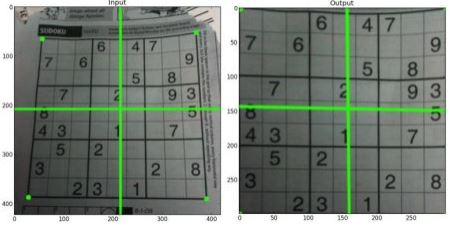一个例子如下：

import cv2
import numpy as np
import matplotlib.pyplot as plt

rows,cols = img.shape[:2]
pts1 = np.float32([[56,65],[238,52],[28,237],[239,240]])
pts2 = np.float32([[0,0],[200,0],[0,200],[200,200]])
M = cv2.getPerspectiveTransform(pts1,pts2)
res = cv2.warpPerspective(img,M,(200,200))
plt.subplot(121)
plt.imshow(img)
plt.subplot(122)
plt.imshow(res)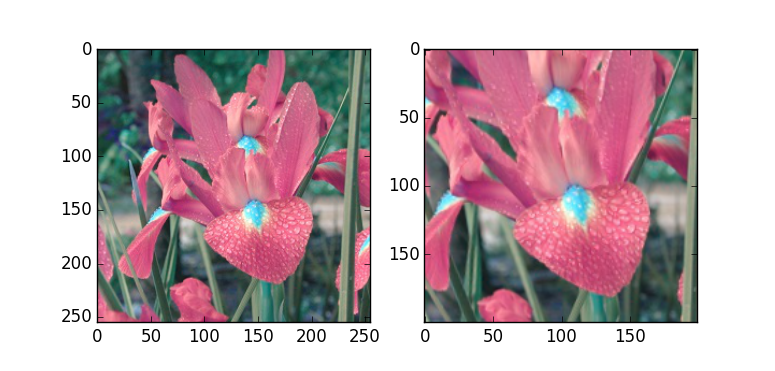展开全文图像处理
• python-opencv实现图像仿射变换

千次阅读 2019-05-29 15:28:19
python-opencv实现图像仿射变换的代码： 所需实现的功能： 对一幅二值图像中值为255的轮廓内的部分进行放大缩小操作，需保证中心点位置不变。 groundtruth = cv2.imread(groundtruth_path)[:, :, 0] h1, w1 = ...
• 仿射变换： 透视变换： 原理和C++实现请看我的另外一篇文章： https://blog.csdn.net/RayChiu757374816/article/details/120174397 仿射变换： 原图： 测试代码： import cv2 import numpy as np import ...opencv python
• python仿射变换

千次阅读 2019-05-29 15:15:57
记录学习Python Computer Vision的过程 第三次 Homography（单应性变换） 图像变换的方法很多，单应性变换是其中一种方法，单应性变换会涉及到单应性矩阵。单应性变换的目标是通过给定的几个点（通常是4对点）来得到...
• 图像img的像素为534*300，img.shape = (300, 534, 3)。 需要引入： import cv2 as cv import numpy as np ...img.shape得到的(300, 534, 3)，前者300是高度，y轴的值...cv2.warpAffine() 仿射变换（从二维坐标到二维python opencv 计算机视觉 图像处理
• 5.我们利用这个角度得到一个旋转的矩形（仿射变换所需要的） 6.使用仿射变换得到矫正后的图像 1.得到灰度图像 //读取图像 src= cv.imread('imageTextR.png') //cv.imshow('src',src) //cvtColor函数用于转换色彩...opencv 计算机视觉 python
• 在前面实现了平移和缩放，还有一种常用的坐标变换，那就是旋转。比如拍摄的照片上传到电脑里，再打开时发现人的头在下面脚在上，这样肯定看不了，那么就需要把这个照片旋转180度才可以接受。由于旋转变换，都是绕着...
• 写文章不易，如果您觉得此文对您有所帮助，请帮忙点赞、评论、收藏，感谢您！一. 仿射变换介绍：二. 仿射变换 公式：仿射变换过程，(x,y)表示原图像中的... python实现仿射变换——图像平移1 importcv22 importnumpy...
• 本文是老猿关于仿射变换的原理、概念以及OpenCV-Python实现额的导览式文章，通过本文作为入口，读者可以非常快速的理解仿射变换的相关知识以及开发应用。仿射变换 编程语言 程序开发 图形图像处理
• 本文介绍了老猿在使用OpenCV-Python进行仿射变换开发中遇到的坑，这些问题可能大家或多或少会碰到，这些问题涉及基本知识的理解、官方资料的坑等方面，希望能帮助到大家。图形图像处理 仿射变换 编程语言 程序开发
• 对于仿射密码算法过程这里不做过多讲解，请自行参照教材或者网络资料。这里需要注意的是，默认为...下面是用python实现的加解密过程： 密钥为k=（11,4） （1）加密过程 （2）解密过程，密钥k的逆元求出后直接带到公式python 加密 算法 仿射密码
• 利用Python实现图像的仿射变换   仿射变换通常用于图像的几何矫正、图像配准   在透视映射下，物体的形状会失真。例如，虽然原本矩形的窗户、书本，由于成像角度的原因，最终呈现在图像上，便会是另外一种不太...图像变换 仿射变换 图像校正 Python opencv
• python+opencv三点仿射变换

千次阅读 2019-02-26 15:56:27
一张图来表明要实现的功能： 说明：将一张正正方方的矩形图转换成平行四边形(甚至不规则四边形)的操作。...三个点的坐标就是6个数字，我们把6个数字设置成自己想要的位置，就可以实现三点仿射变换。我...opencv python
• 1.4 Python图像的坐标变换-仿射变换 文章目录1.4 Python图像的坐标变换-仿射变换1 算法原理2 代码3 效果 1 算法原理 图像的几何变换，也就是矩阵乘法。 通过仿射变换将图片中的每个像素点按照一定的规律映射到新的...python opencv 计算机视觉
• 仿射变换opencv提供了函数cv2.getAffineTransform()来创建一个2*3的矩阵，该矩阵传递给cv2.warpAffine()。该函数语法格式为：retval = cv.getAffineTransform(src, dst)'''src：输入图像的三个点坐标dst：输出图像的...
• 本节介绍了仿射变换的概念、类型、基本仿射变换矩阵、OpenCV-Python仿射变换相关的主要函数及语法说明，并提供了两种不同方式实现的图像旋转和任选三个点将圈定子图放大的示例。通过阅读相关内容可以有助于大家...图形图像处理 仿射变换 编程语言 程序开发
• 对图像块应用仿射变换，我们将其称为图像扭曲（或者仿射扭曲）。该操作不仅经常应用在计算机图形学中，而且经常出现在计算机视觉算法中。 一、仿射变换原理 仿射变换能够保持图像的“平直性”，包括旋转，缩放，平移...
• import cv2 import numpy as np from matplotlib import pyplot as plt img = cv2.imread('pic3.PNG') cv2.imshow('img', img) cv2.waitKey(0) cv2.destroyAllWindows() rows, cols = img.shape[:2] ...
• 仿射密码思路：1、加解密公式：2、构造对应字典：3、代码实现构造字典，建立映射关系：# 构造字典，'A' --> 0 ...def char_2_num(x):list_s = []list_num = []for i in range(26):c = chr(i + 65)list_s.append(c...仿射密码python...

python实现仿射变换python 订阅